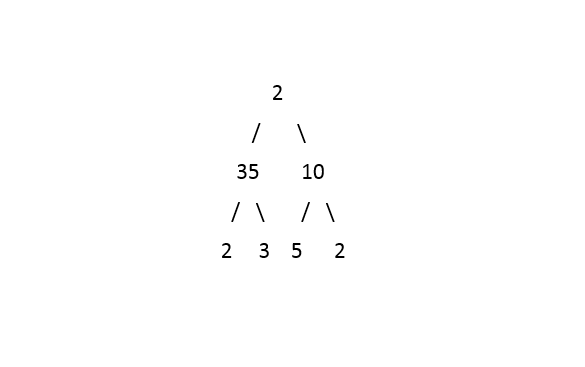New update is available. Click here to update.
Last Updated: 27 Jul, 2020
##### Top View
Moderate
Problem statement#### Given a binary tree. Print the Top View of Binary Tree. Print the nodes from left to right order.

##### Example:
``````Input:
````````````Output: 2 35 2 10 2
``````
##### Input format :
``````The first line contains an Integer 't' which denotes the number of test cases or queries to be run. Then the test cases follow.

The first line of input contains the elements of the tree in the level order form separated by a single space.
If any node does not have a left or right child, take -1 in its place. Refer to the example below.

Example:

Elements are in the level order form. The input consists of values of nodes separated by a single space in a single line. In case a node is null, we take -1 on its place.

For example, the input for the tree depicted in the below image would be :
````````````1
2 3
4 -1 5 6
-1 7 -1 -1 -1 -1
-1 -1

Explanation :
Level 1 :
The root node of the tree is 1

Level 2 :
Left child of 1 = 2
Right child of 1 = 3

Level 3 :
Left child of 2 = 4
Right child of 2 = null (-1)
Left child of 3 = 5
Right child of 3 = 6

Level 4 :
Left child of 4 = null (-1)
Right child of 4 = 7
Left child of 5 = null (-1)
Right child of 5 = null (-1)
Left child of 6 = null (-1)
Right child of 6 = null (-1)

Level 5 :
Left child of 7 = null (-1)
Right child of 7 = null (-1)

The first not-null node (of the previous level) is treated as the parent of the first two nodes of the current level. The second not-null node (of the previous level) is treated as the parent node for the next two nodes of the current level and so on.

The input ends when all nodes at the last level are null (-1).
``````
##### Note :
``````The above format was just to provide clarity on how the input is formed for a given tree.

The sequence will be put together in a single line separated by a single space. Hence, for the above depicted tree, the input will be given as:

1 2 3 4 -1 5 6 -1 7 -1 -1 -1 -1 -1 -1
``````
##### Output Format :
``````For each test case, print the Top View of Tree from left to right.

Output for every test case will be printed in a separate line.
``````
##### Constraint :
``````1 <= T <= 100
1 <= N <= 1000

Time Limit : 1 sec
``````Approaches

## 01ApproachBefore looking at the approach, let's define a couple of terms; the level of a node and the distance of a node. The level of a node means the depth of the node with respect to the root node. So, the level of the root node will be 0, its children's would be 1, and so on. To generalize, if the level of a node is ‘x’, then the level of its children would be ‘x + 1’.

The distance of a node is a measure of how far a node is from the root node in the horizontal direction. It is negative in the left direction and positive in the right direction. For example, the distance for the root node is 0, the distance for root's left child is -1 and for its right child is 1. To generalize, if the distance of a node is ‘x’, then the distance for its left child would be ‘x - 1’ and for its right child would be ‘x + 1’.

The following image will give an example of a tree with the level and distance of all nodes marked in the format ('distance', ‘level’)